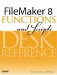# Seconds()

Category: Time

 Syntax: Seconds ( time )

Parameters:

timeAny valid time value or expression that returns a valid time value.

Data type returned: Number

Description:

The Seconds() function returns an integer representing the number of seconds specified by the time parameter.

This function always returns a value from 0 to 59.

If you want to express the output of this function as a two-character string rather than as an integer (for example, 03 rather than 3), use the following formula:

``` Right ("00" & Seconds (Time); 2)
```

If the time parameter has a seconds value greater than 59, the Seconds() function returns the Mod-60 result of that value. For instance, Seconds ("12:42:87") returns 27. Note that the "overflow" of the seconds value is applied to the minutes value: Minute ("12:42:87") returns 43.

Examples:

Function

Results

Seconds ("10:45:20")

Returns 20.

Seconds ("12:15 am")

Returns 0.FileMaker 8 Functions and Scripts Desk Reference
ISBN: 0789735113
EAN: 2147483647
Year: 2004
Pages: 352